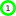# Java: Summary - Data

##Basic Java

Basic types to know: int, double, String, boolean, and char.

## Classes

There are 8 primitive types: `boolean` (true and false); `char` (Unicode characters); `byte`, `short`, `int`, and `long` (integers); `float` and `double` (floating point). The most commonly used pre-defined object type is String. Primitive types have corresponding "wrapper" class whose objects are immutable (values can not be changed).

Autoboxing. Conversion between the primitive and the wrapper classes is largely automatic as of Java 5.

## Integer classes

The integer types byte, short, int, and long are stored as two's complement, signed binary integers. char, which is technically also an integer type, is stored as an unsigned binary number. Expressions are computed as ints, so a cast is needed to store in a smaller type.
 Type Bytes Range Literals byte 1 -128..+127 none short 2 -32,768..+32,767 none int 4 -2,147,483,648..+2,147,483,647 23, 0xAF long 8 -9,223,372,036,854,775,808.. 23L, 0xAFL
Operators: arithmetic, comparison, bitwise, assignment.
Wrapper classes Integer (not Int), Short, Byte, Long contain utility methods, eg, `Integer.parseInt(s)`, `MAX_VALUE`, `MIN_VALUE`, ...

Math class has many useful utility methods.

java.math.BigInteger is useful for arithmetic on unbounded integers.

Several other integer classes have limited or special utility: Number, AtomicInteger, and AtomicLong.

## Floating-point classes

The floating-point types, float and double, are stored in IEEE-754 format. Calculations may produce NaN (Not a Number) or +/- infinity. Calculations are done as doubles, so a cast is needed to store in a float.
 Type Bytes Range Accuracy Literals float 4 -3.4E38..+3.4E38 6-7 digits 3.14F 6.02e23F double 8 -1.7E308..+1.7E308 14-15 digits 3.14 6.02e23
Operators: arithmetic, comparison, assignment.
Wrapper classes: Double and Float contain utility methods (eg, `Double.parseDouble(s)`), `MAX_VALUE`, `MIN_VALUE`, ...

Math class has many useful utility methods.

java.math.BigDecimal for arithmetic on unbounded floating-point numbers.

Special utility: Number.

## Boolean class

boolean has values true or false.
Operators: logical, ==, !=, assignment.
Boolean: wrapper class with little utility.

## Character, String, ... classes

char type is a Unicode character stored as an unsigned number in two bytes (range 0..65,535 or '\u0000'..'\uFFFF'). char is an integer type.
char literals
• 'A' (single character)
• Unicode '\uxxxx' where x is a hexadecimal digit. Eg '\u0041'
• Octal '\nnn' where n is an octal digit.
• Escape combinations: '\n' newline, '\\' backslash, '\'' single quote, '\"' double quote, '\r' carriage return, '\t' tab, '\b' backspace, '\f' form feed.
Character wrapper class contains useful methods for working with characters.

String immutable 0 or more characters.

java.util.StringBuilder (as of Java 5) and java.util.StringBuffer (slower than StringBuilder because it's synchronized) used for dynamically building or modifying strings.

java.util.regex.Pattern and java.util.regex.Matcher used for regular expression matching. java.util.StringTokenizer is earlier class to break strings into "tokens".

CharSequence is basic character interface.

2005 Fred Swartz